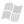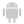Home |Sign in | EnglishHelpWLanguageWLanguage functionsControls, pages and windowsPivot Table functionsPrefix syntaxPVTCalculateAllPresentationExampleHow is the calculation performed?Progress of calculationRelated examplesSee alsoConstants for managing Pivot Table controlsPVTAxisXYPVTCalculateAllPVTCalculateUpdatePVTCollapsePVTCollapseAllPVTCompareDateRangePVTExpandPVTExpandAllPVTFilterPVTHeaderValuePVTInfoXYPVTListPositionHeaderPVTLoadPVTSavePVTSelectPVTSelectOccurrencePVTToExcelPivot Table control management functionspvtPosition
• How is the calculation performed?
• Progress of calculation
WINDEVWEBDEVWINDEV MobileOthersPVTCalculateAll (Function)
In french: TCDCalculeTout
Entirely calculates or recalculates a Pivot Table control. Caution: this operation may take quite a long time.
Example
IF YesNo("Do you want to entirely recalculate the Pivot Table control?") THEN
PVTCalculateAll(PVT_Sales)
END
Syntax
PVTCalculateAll(<Pivot Table control>)
<Pivot Table control>: Control name
Name of Pivot Table control to calculate or recalculate.
Remarks

How is the calculation performed?

The calculation is performed by browsing all the necessary records. This calculation can be quite long according to the size of the database. For example, a calculation performed on one million records can take several minutes.
• The content of the Pivot Table control must be entirely recalculated:
• When adding new items to display.
• When modifying the filtering functions.
• When modifying the source data of calculation.
• The content of the Pivot Table control does not need to be entirely recalculated:
• When using a filter on a dimension that is already calculated.
• When changing dimension.
• When expanding or collapsing a dimension (to view the trimesters instead of the years for example).

Progress of calculation

• A progress bar is displayed during the calculation of the Pivot Table control.
•Versions 20 and later
The user has the ability to cancel the calculation via a "Cancel" button.To find out whether the calculation was entirely performed, use the ErrorOccurred variable.
Example:

PVTCalculateAll(PVT_Statistics)
IF ErrorOccurred = True THEN
SWITCH Dialog("Do you want to cancel the current process?")
// Cancel
CASE 1
Close()
// Continue
CASE 0
END
ENDNew in version 20
The user has the ability to cancel the calculation via a "Cancel" button.To find out whether the calculation was entirely performed, use the ErrorOccurred variable.
Example:

PVTCalculateAll(PVT_Statistics)
IF ErrorOccurred = True THEN
SWITCH Dialog("Do you want to cancel the current process?")
// Cancel
CASE 1
Close()
// Continue
CASE 0
END
END
The user has the ability to cancel the calculation via a "Cancel" button.To find out whether the calculation was entirely performed, use the ErrorOccurred variable.
Example:

PVTCalculateAll(PVT_Statistics)
IF ErrorOccurred = True THEN
SWITCH Dialog("Do you want to cancel the current process?")
// Cancel
CASE 1
Close()
// Continue
CASE 0Training (WINDEV): WD PivotTable [ + ] This example presents the use of the Pivot Table control.This Pivot Table control is used to display dynamic statistics while grouping the data.These statistics are calculated form the database.